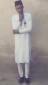# Bernoulli's differential equationHello ,please someone should help with out with these Diffrential equations
a. xdy + ydx = x^3 y^6 dx
b. cosysin2xdx +(cos^2y - cos^x)dy = 0### Part (a) xdy + ydx = x^3 y^6

Part (a) xdy + ydx = x^3 y^6 dx
$x \, dy + y \, dx = x^3 y^6 \, dx$

$dy + \dfrac{1}{x}y \, dx = x^2 y^6 \, dx$

$P = \dfrac{1}{x}$

$Q = x^2$

$n = 6$

$(1 - n) = -5$

$z = y^{1 - n} = y^{-5}$

Integrating Factor
\begin{align} \displaystyle u & = e^{(1 - n)\int P\,dx} \\ & = e^{-5\int \frac{1}{x}\,dx} \\ & = e^{-5 \ln x} \\ & = e^{\ln x^{-5}} \\ & = x^{-5} \end{align}

Thus,
$\displaystyle zu = (1 - n) \int Qu \, dx + C$

$\displaystyle y^{-5}x^{-5} = -5 \int x^2 (x^{-5}) \, dx + C$

$\displaystyle x^{-5}y^{-5} = -5 \int x^{-3} \, dx + C$

$x^{-5}y^{-5} = -5 \left( \dfrac{x^{-2}}{-2} \right) + C$

$x^{-5}y^{-5} = \frac{5}{2} x^{-2} + C$

• Mathematics inside the configured delimiters is rendered by MathJax. The default math delimiters are $$...$$ and $...$ for displayed mathematics, and $...$ and $...$ for in-line mathematics.